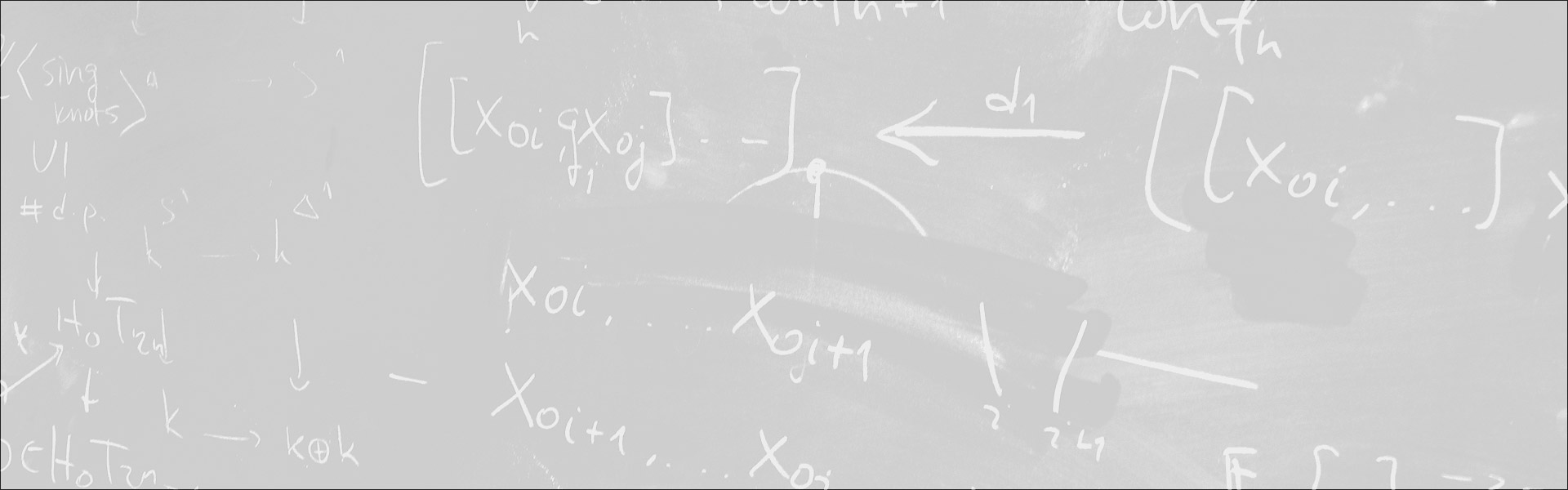Designed and built with care, filled with creative elements

Top# Effective isotrivial Mordell-Lang in positive characteristic

/  Évènements• Cet évènement est passé

## Effective isotrivial Mordell-Lang in positive characteristic

The Mordell-Lang conjecture (now a theorem, proved by Faltings, Vojta, McQuillan,…) asserts that if G is a semiabelian variety G defined over an algebraically closed field of characteristic zero, X is a subvariety of G, and Γ is a finite rank subgroup of G, then X ∩ Γ is a finite union of cosets of Γ. In positive characteristic, the naive translation of this theorem does not hold, however Hrushovski, using model theoretic techniques, showed that in some sense all counterexamples arise from semiabelian varieties defined over finite fields (the isotrivial case). This was later refined by Moosa and Scanlon, who showed in the isotrivial case that the intersection of a subvariety of a semiabelian variety G with a finitely generated subgroup Γ of G that is invariant under the Frobenius endomorphism F: G → G is a finite union of sets of the form S+A, where A is a subgroup of Γ and S is a sum of orbits under the map F. We show how how one can use finite-state automata to give a concrete description of these intersections Γ ∩ X in the isotrivial setting, by constructing a finite machine that identifies all points in the intersection. In particular, this allows us to give decision procedures for answering questions such as: is X ∩ Γ empty? finite? does it contain a coset of an infinite subgroup? In addition, we are able to read off coarse asymptotic estimates for the number of points of height ≤ H in the intersection from the machine. This is joint work with Dragos Ghioca and Rahim Moosa.

- Séminaire Géométrie et théorie des modèles

#### Détails :

Orateur / Oratrice : Jason Bell
Date : 26 mars 2021
Horaire : 15h00 - 16h20
Lieu : Zoom